# SAT Math Multiple Choice Question 996: Answer and Explanation

### Test Information

Question: 996

16.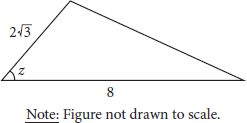If the area of the triangle shown above is 12 square inches, what is the value ofcos z?

• A.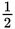• B.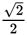• C.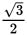• D. 1

Explanation:

A

Difficulty: Hard

Category: Additional Topics in Math / Trigonometry

Strategic Advice: Sometimes, it is necessary to draw in the height of a triangle to get an idea of which side lengths you need. Use the information given about the area of the triangle to find its height.

Getting to the Answer: Find the height of the triangle and add it to the figure: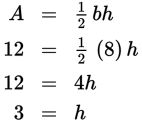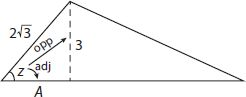Next, use the Pythagorean theorem to find the missing side of the right triangle that contains z:Finally, use SOH CAH TOA: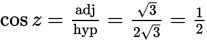. This means (A) is correct.